MORE IN Engineering Mechanics (EM)
SPPU First Year Engineering (Semester 2)
Engineering Mechanics (EM)
December 2013
Total marks: --
Total time: --
INSTRUCTIONS
(1) Assume appropriate data and state your reasons
(2) Marks are given to the right of every question
(3) Draw neat diagrams wherever necessary

Answer any one question from Q1 & Q2
1 (a) The resultant of two forces P and Q is 1200 N horizontally leftward. Determine the force Q and corresponding angle ? for the system of force s as shown in Fig. 1a.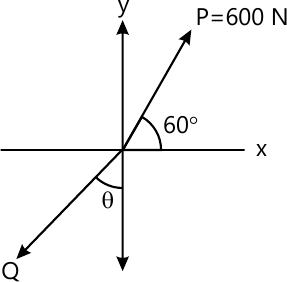4 M
1 (b) The tanker is pulled with constant acceleration of 0.001 m/s2 using cable that makes an angle of 10° with the horizontal as shown in Fig. 1b. If the force in the cable is 45.694 KN, determine the mass of tanker using Newton's 2nd law of motion.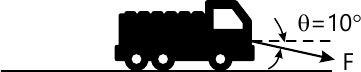4 M
1 (c) An outdoor track is 126 m in diameter. A runner increases her speed at a constant rate from 4.2 to 7.2 m/s over a distance of 28.5 m. Determine the total acceleration of the runner 2 s after she begins to increase her speed.
4 M
1 (d) A 2 kg stone is dropped from a height h and strike the ground with a velocity of 24 m/s. Find the kinetic energy of the stone as it strikes the ground and the height h from which it was dropped using work energy method.
4 M

2 (a) Determine the y coordinate of centroid of the shaded area as shown in Fig. 2a.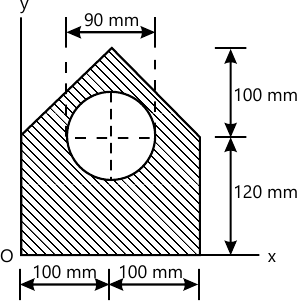4 M
2 (b) A sphere is fired into a medium with a initial speed of 27 m/s. If it experience a deceleration a=(-6t) m/s2, where t is in seconds, determine the distance traveled before it stop.
4 M
2 (c) The small 0.6 kg block slides on a smooth circular path of radius 3 m in the vertical plane. If the speed of the block is 5 m/s as it passes point A and 4 m/s as it passes point B, determine the normal force exerted on the block by the surface at each of these location. Refer Fig. 2 c.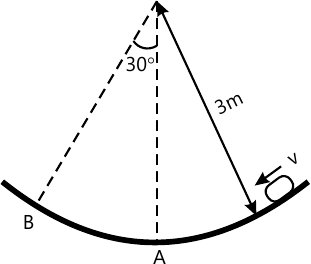4 M
2 (d) A railroad car having a mass of 15 Mg is coasting at 1.5 m/s on a horizontal track. At the same time another car having a mass of 12 Mg is coasting at 0.75 m/s in the opposite direction. If the car meet and couple together, determine the speed of both cars just after the coupling.
4 M

Answer any one question from Q3 & Q4
3 (a) Determine the magnitude of F1 and F2 so that the particle is in equilibrium. Refer Fig. 3a.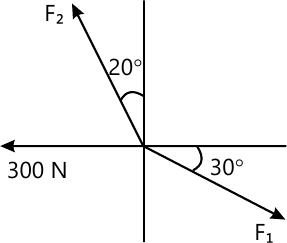6 M
3 (b) Determine the reactions at support for the beam loaded and supported as shown in Fig. 3b.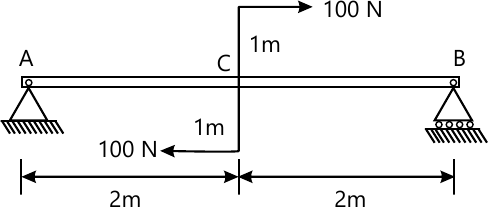5 M
3 (c) A concrete foundation mat of 5 m radius supports four equally spaced columns, each of which is located 4 m from the centre of the mat. Determine the magnitude and point of application of the resultant of the four loads as shown in Fig. 3 c.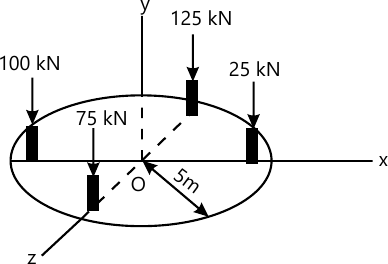6 M

4 (a) A 300 mm wooden beam weighing 540N is supported by a pin and bracket at A and by cable BC. Find the reaction at A and tension in cable BC. Refer Fig. 4 a.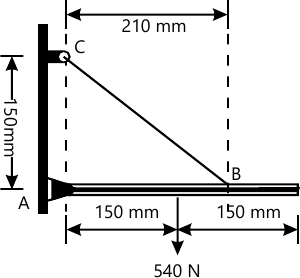6 M
4 (b) A rectangular plate is supported by three cables at A as shown in Fig. 4 b. Knowing that the tension in cable AD is 120 N, determine the weight of the plate.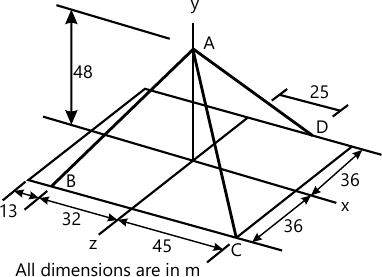6 M
4 (c) State and explain Two Force and Three Force Principle for equilibrium with sketches.
5 M

Answer any one question from Q5 & Q6
5 (a) Determine the magnitude and nature of forces in the members AB, AH and GC of the truss loaded and supported as shown in Fig. 5 a.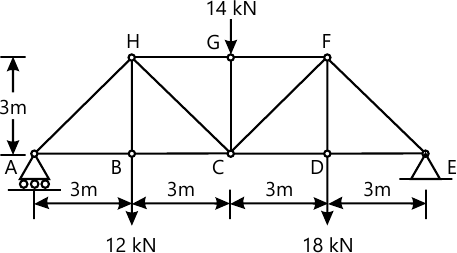6 M
5 (b) The 15 m ladder has a uniform weight of 80 N and rest against the smooth wall at B as shown in Fig. 5 b. If the coefficient of static friction between ladder and floor is ?s=0.4, determine the smallest angle ? at which the ladder will not slip.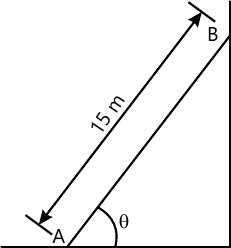6 M
5 (c) Define angle of repose, angle of friction, coefficient of friction and cone of friction with sketches.
5 M

6 (a) Determine the forces in number of HG, HC and BC in the truss as shown in Fig. 5a. Use method of section.
6 M
6 (b) Determine the horizontal force P needed to just moving the 300 N block up the plane. Take ?s=0.3 and refer Fig. 6b.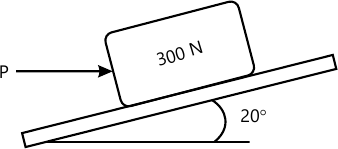5 M
6 (c) Two loads are suspended as shown in Fig. 6C from cable ABCD. Knowing the dc=0.75 m and db=1.125 m, determine the component of reaction at A and maximum tension in the cable.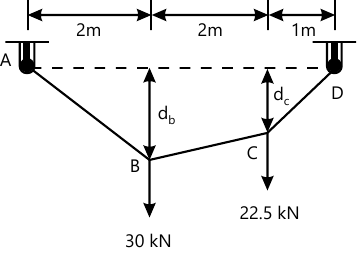6 M

More question papers from Engineering Mechanics (EM)